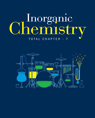×#### Thank you for registering.

One of our academic counsellors will contact you within 1 working day.

Click to Chat

1800-1023-196

+91-120-4616500

CART 0

• 0

MY CART (5)

Use Coupon: CART20 and get 20% off on all online Study Material

ITEM
DETAILS
MRP
DISCOUNT
FINAL PRICE
Total Price: Rs.

There are no items in this cart.
Continue Shopping
```
a compound contains 61%of carbon,11.88% hydrogen and 27.12%oxygen.if the volume density of compound is 59%.calculate the molecular formula.
a compound contains 61%of carbon,11.88% hydrogen and 27.12%oxygen.if the volume density of compound is 59%.calculate the molecular formula.

```
3 years ago

```							Dear student C=61/12=5.08H=11.8/1=11.8O=27/16=1.68Lowest ratio value multiple to all valueC=5.08/1.68=3.02H=11.8/1.68=7.2O=1.68/1.68=1.68Empirical formula is =C3H7O1Empirical formula mass =3×12+7×1+1×16=59n=density /emyrical formula mass =59/59=1Molecular formula =n×emyrical formula =1×C3H7O1=C3H7O
```
one year ago
Think You Can Provide A Better Answer ?

## Other Related Questions on Inorganic Chemistry

View all Questions »### Course Features

• 731 Video Lectures
• Revision Notes
• Previous Year Papers
• Mind Map
• Study Planner
• NCERT Solutions
• Discussion Forum
• Test paper with Video Solution### Course Features

• 54 Video Lectures
• Revision Notes
• Test paper with Video Solution
• Mind Map
• Study Planner
• NCERT Solutions
• Discussion Forum
• Previous Year Exam Questions